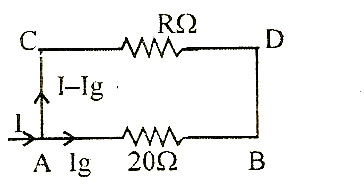# A galvanometer of resistance 20 W gives full scale detlection with a current of 0.004 A. To convert it into an ammeter of range 1 A, the required shunt resistance should be         (d)    0.38 ohm         (b)    0.21 ohm         (c)    0.08 ohm       (d)    0.05 ohm8 years ago

(c)

Maximum current which can pass through galvanometer,

Ig = 0.004 ALet R be the resistance of shunt.

We know potential drop across AB = Potential drop across CD

R (I -Ig) = Ig (20)

Þ R(1- 0.004) = 0.004 × 20 Þ R = 0.08 W